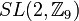# Special linear group:SL(2,Z9)

View a complete list of particular groups (this is a very huge list!)[SHOW MORE]

## Definition

The group, denoted$SL(2,\mathbb{Z}_9)$ or$SL(2,\mathbb{Z}/9\mathbb{Z})$, is defined as the special linear group of degree two over the ring of integers modulo 9.

## Arithmetic functions

Want to compare and contrast arithmetic function values with other groups of the same order? Check out groups of order 648#Arithmetic functions

### Basic arithmetic functions

Function Value Similar groups Explanation for function value
order (number of elements, equivalently, cardinality or size of underlying set) 648 groups with same order As$SL(2,\mathbb{Z}_9)$, where$\mathbb{Z}_9$ is a DVR of length$l = 2$ over a field of size$q = 3$:$q^{3l - 2}(q^2 - 1) = 3^{3(2) - 2}(3^2 - 1) = (81)(8) = 648$
exponent of a group 36 groups with same order and exponent of a group | groups with same exponent of a group PLACEHOLDER FOR INFORMATION TO BE FILLED IN: [SHOW MORE]
nilpotency class -- -- not a nilpotent group
derived length 3 groups with same order and derived length | groups with same derived length PLACEHOLDER FOR INFORMATION TO BE FILLED IN: [SHOW MORE]
Frattini length 2 groups with same order and Frattini length | groups with same Frattini length The Frattini subgroup is the center, which is cyclic of order two.
Fitting length 3 groups with same order and Fitting length | groups with same Fitting length PLACEHOLDER FOR INFORMATION TO BE FILLED IN: [SHOW MORE]

### Arithmetic functions of a counting nature

Function Value Similar groups Explanation for function value
number of conjugacy classes 25 groups with same order and number of conjugacy classes | groups with same number of conjugacy classes As$SL(2,R)$,$R$ a DVR of length$l = 2$ over a field of size$q$,$q$ odd:$q^l + 4 \frac{q^l - 1}{q - 1} = 3^2 + 4 (8)/2 = 9 + 16 = 25$ (note that for$l = 2$, the formula simplifies to$(q + 2)^2$)
See element structure of special linear group of degree two over a finite discrete valuation ring, element structure of special linear group:SL(2,Z9)
number of conjugacy classes of subgroups 48 groups with same order and number of conjugacy classes of subgroups | groups with same number of conjugacy classes of subgroups See subgroup structure of special linear group:SL(2,Z9)
number of subgroups 456 groups with same order and number of subgroups | groups with same number of subgroups See subgroup structure of special linear group:SL(2,Z9)

## GAP implementation

### Group ID

This finite group has order 648 and has ID 641 among the groups of order 648 in GAP's SmallGroup library. For context, there are groups of order 648. It can thus be defined using GAP's SmallGroup function as:

SmallGroup(648,641)

For instance, we can use the following assignment in GAP to create the group and name it$G$:

gap> G := SmallGroup(648,641);

Conversely, to check whether a given group$G$ is in fact the group we want, we can use GAP's IdGroup function:

IdGroup(G) = [648,641]

or just do:

IdGroup(G)

to have GAP output the group ID, that we can then compare to what we want.

### Other descriptions

Description Functions used
SL(2,ZmodnZ(9)) SL, ZmodnZ